Next: Pairing-plus-quadrupole model Up: Deformed shell (finite non-degenerate Previous: Deformed shell (finite non-degenerate

### Second-order perturbation results

Following Sec. 3.1, one can derive analytic expressions for the binding energy filters which should be valid in the limiting cases of weak and strong pairing. Particularly simple are the weak-pairing expressions. Namely, Eqs. (21) yield: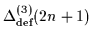=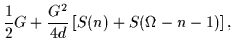(57)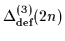=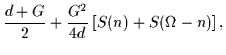(58)

where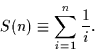(59)

Using Eq. (23), the single-particle splitting filters are: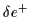=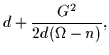(60)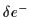=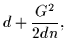(61)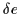=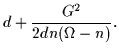(62)

Note that in this case, the single-particle splitting filters (60) are always greater than the original spacing d. In particular,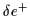increases with n,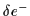decreases with n, andhas a minimum in the middle of the shell.

In the strong-pairing limit of the equidistant-level model, the zero-order expressions for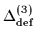andare given by Eqs. (40) and (41). The corresponding higher-order corrections can be easily derived using Eqs. (27)-(36) after noting that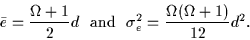(63)

Figure 8 shows the accuracy of the second-order expressions for the binding energies in the equidistant-level model with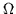=16 in the weak-pairing (left) and strong-pairing (right) limits. It is seen that, for large numbers of particles, the low-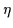expansion is fairly accurate even for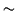0.4. Also the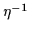expansion seems to work very well even for large values of d/G. different verticalNext: Pairing-plus-quadrupole model Up: Deformed shell (finite non-degenerate Previous: Deformed shell (finite non-degenerate
Jacek Dobaczewski
2000-03-09